• java保留两位小数问题： 一： 四舍五入(四舍五入形式保留两位小数，注意模式ROUND_HALF_UP) double f = 2345.2345; BigDecimal b = new BigDecimal(f); double f1 = b.setScale(2, BigDecimal.ROUND_HALF_UP).double...
java保留两位小数问题： 一： 四舍五入(四舍五入形式保留两位小数，注意模式ROUND_HALF_UP) double f = 2345.2345; BigDecimal b = new BigDecimal(f); double f1 = b.setScale(2, BigDecimal.ROUND_HALF_UP).doubleValue(); 保留两位小数 二： double f = 2345.2345; java.text.DecimalFormat df =new java.text.DecimalFormat("#.00"); df.format(f); 例：new java.text.DecimalFormat("#.00").format(2.1234567) #.00 表示两位小数 #.0000四位小数 以此类推… 三： double d = 2.1234567; String result = String .format("%.2f"); %.2f %. 表示 小数点前任意位数 2 表示两位小数 格式后的结果为f 表示浮点型 四： NumberFormat ddf1=NumberFormat.getNumberInstance() ; void setMaximumFractionDigits(int digits) digits 显示的数字位数 为格式化对象设定小数点后的显示的最多位,显示的最后位是舍入的 import java.text.* ; import java.math.* ; class TT { public static void main(String args[]) { double x=2.1234567; NumberFormat ddf1=NumberFormat.getNumberInstance() ; ddf1.setMaximumFractionDigits(2); String s= ddf1.format(x) ; System.out.print(s); } } 二 、BigDecimal 的加减乘除使用 BigDecimal bignum1 = new BigDecimal(“10”); BigDecimal bignum2 = new BigDecimal(“5”); BigDecimal bignum3 = null; //加法 bignum3 = bignum1.add(bignum2); System.out.println(“和 是：” + bignum3);
//减法 bignum3 = bignum1.subtract(bignum2); System.out.println(“差 是：” + bignum3);
//乘法 bignum3 = bignum1.multiply(bignum2); System.out.println(“积 是：” + bignum3);
//除法 bignum3 = bignum1.divide(bignum2); System.out.println(“商 是：” + bignum3);
展开全文• php中文网最新课程每日17准时技术干货分享这篇文章主要介绍了PHP保留两位小数的几种方法，在一些商品价格上也经常遇到这样的需求，今天小编给大家分享的实例代码,需要的朋友可以参考下代码如下所示：$num = 10....php中文网最新课程 每日17点准时技术干货分享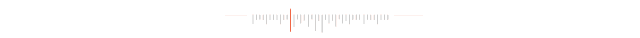这篇文章主要介绍了PHP保留两位小数的几种方法，在一些商品价格上也经常遇到这样的需求，今天小编给大家分享的实例代码,需要的朋友可以参考下 代码如下所示： $num = 10.4567;    //第一种：利用round()对浮点数进行四舍五入  echo round($num,2); //10.46 //第二种：利用sprintf格式化字符串$format_num = sprintf("%.2f",$num); echo$format_num; //10.46  //第三种：利用千位分组来格式化数字的函数number_format()  echo number_format($num, 2); //10.46 //或者如下 echo number_format($num, 2, '.', ''); //10/46
下面看下PHP中对一些商品的价格计算或价格的的展示，需要精确到小数点后的两位数字，也就是我们平时RMB中的分的单位。
那在PHP中如何展示商品的价格，并保留到分的单位的呢？
PHP number_format() 函数
number_format()：函数可以通过千位分组的形式来格式化数字。
语法：
number_format(number,decimals,decimalpoint,separator)
参数：
number：必需。要格式化的数字。
decimals：可选。规定多少个小数。
decimalpoint：可选。规定用作小数点的字符串。
separator：可选。规定用作千位分隔符的字符串。
例：PHP商品价格以元为单位，保留两位小数
<?php $a = 10;echo number_format($a,'2');$b = 1000000;echo number_format($b,'2');$c = 5458.5684;echo number_format($c,'2');$d = '1254.8963';echo number_format($d,'2');$e = '88.9643';echo number_format($e,'2');?>
输出结果：
10.001,000,000.005,458.571,254.9088.96
以上示例总结：
1、无论是数字类型或是字符串类型的数字，都可以被 number_format() 函数操作
2、number_format() 在操作不含有小数的数字时，如果设置了有多小个小数，会以 0 的形式补充。
3、如果操作带有多少小数的数字，会以四舍五入的方式进行最近一位的取值
4、如果不设置 number_format() 第三个和第四个参数，整数部分如果大于3位，那么从小数点左边向右开始，每三位都用','号分割-END-
声明：本文选自「 php中文网 」，搜索「 phpcnnew 」即可关注！
▼


展开全文• // 四舍五入 BigDecimal value = new BigDecimal(object.toString()).setScale(2,BigDecimal.ROUND_HALF_UP);... // 不足两位小数补0 DecimalFormat decimalFormat = new DecimalFormat("0.00#"); ...
  // 四舍五入
BigDecimal value = new BigDecimal(object.toString()).setScale(2,BigDecimal.ROUND_HALF_UP);
// 不足两位小数补0
DecimalFormat decimalFormat = new DecimalFormat("0.00#");
String strVal = decimalFormat.format(value);

展开全文• 2、BigDecimal初始化赋值3、BigDecimal的加减乘除运算4、BigDecimal比较大小5、BigDecimal保留两位小数及舍入模式6、BigDecimal其他方法及常量 1、为什么要用BigDecimal ？ 工作中我们通过浮点数进行运算时，好像时...


文章目录
1、为什么要用BigDecimal ？2、BigDecimal初始化赋值3、BigDecimal的加减乘除运算4、BigDecimal比较大小5、BigDecimal保留两位小数及舍入模式6、BigDecimal其他方法及常量

1、为什么要用BigDecimal ？
工作中我们通过浮点数进行运算时，好像时不时的会出现一些小误差。例如：
public static void main(String[] args) {
System.out.println(1.9 - 1.2);
System.out.println(1.9 - 1.5);
System.out.println(100 - 99.8);
}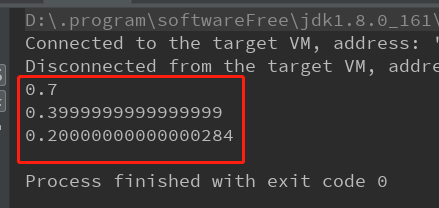大致搜了一下原因，网上说我们的计算机是二进制的，而浮点数是没有办法通过二进制精准的表示出来。 也就导致在运算的时候，float类型和double类型很容易丢失精度。 所以在开发中，如果我们需要精确计算的结果，可以使用java.math包中提供的BigDecimal类来进行操作。
2、BigDecimal初始化赋值
方法类型描述public BigDecimal(int val)构造函数int类型的值生成BigDecimal对象public BigDecimal(long val)构造函数long类型的值生成BigDecimal对象public BigDecimal(String val)静态方法String类型的值转换为BigDecimal类型public static BigDecimal valueOf(double val)静态方法double类型的值转换为BigDecimal类型public static BigDecimal valueOf(long val)静态方法long类型（包含int类型）的值转换为BigDecimal类型
代码示例：
BigDecimal b = new BigDecimal("33");
BigDecimal c = BigDecimal.valueOf(4.7);

注意：不建议使用public BigDecimal(double val)方式初始化值，编码时idea提示禁止使用构造方法BigDecimal(double)，描述如下：
使用了new BigDecimal(double)构造函数 less... (Ctrl+F1)
Inspection info:
禁止使用构造方法BigDecimal(double)的方式把double值转化为BigDecimal对象 说明：反编译出的字节码文件显示每次循环都会new出一个StringBuilder对象，然后进行append操作，最后通过toString方法返回String对象，造成内存资源浪费。

Negative example(不建议使用):
BigDecimal good1 = new BigDecimal(0.1);

Positive example(建议使用):
BigDecimal good1 = new BigDecimal("0.1");
BigDecimal good2 = BigDecimal.valueOf(0.1);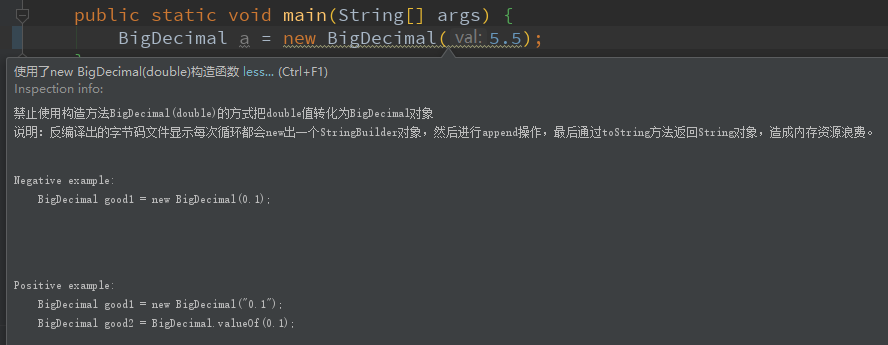3、BigDecimal的加减乘除运算
运算法则对应方法加法public BigDecimal add(BigDecimal value)减法public BigDecimal subtract(BigDecimal value)乘法public BigDecimal multiply(BigDecimal value)除法public BigDecimal divide(BigDecimal value)
代码示例：
public static void main(String[] args) {
System.out.println("计算减法: " + BigDecimal.valueOf(1.9).subtract(BigDecimal.valueOf(1.5)));
System.out.println("计算乘法: " + BigDecimal.valueOf(1.9).multiply(BigDecimal.valueOf(0.2)));
System.out.println("计算除法: " + BigDecimal.valueOf(1.9).divide(BigDecimal.valueOf(0.2)));
}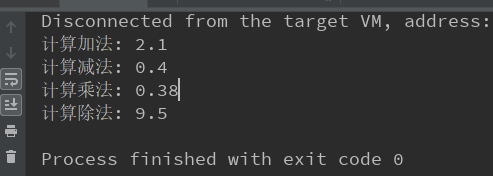注意1：BigDecimal的运算结果都是返回了一个新的BigDecimal对象，并不是在原有的对象上进行操作。
public static void main(String[] args) {
BigDecimal a = BigDecimal.valueOf(5);
System.out.println("a的地址：" + System.identityHashCode(a));
System.out.println("计算后a的地址：" + System.identityHashCode(a));
}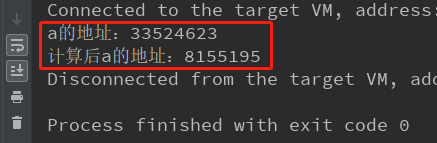注意2：使用divide除法函数除不尽，出现无线循环小数的时候，就需要使用另外精确的小数位数以及舍入模式，不然会出现报错。例如：
public static void main(String[] args) {
BigDecimal a = BigDecimal.valueOf(10), b = BigDecimal.valueOf(3);
System.out.println(a.divide(b));
}

// 该程序运行会出现以下错误
Exception in thread "main" java.lang.ArithmeticException: Non-terminating decimal expansion; no exact representable decimal result.
at java.math.BigDecimal.divide(BigDecimal.java:1690)
at com.fivesix._05_bigdecimal.Demo01.main(Demo01.java:31)

解决方法如下（此处舍入模式使用四舍五入的方式，其他模式在该文章后面有讲解）：
public static void main(String[] args) {
BigDecimal a = BigDecimal.valueOf(10), b = BigDecimal.valueOf(3);
System.out.println(a.divide(b, 3, BigDecimal.ROUND_HALF_UP));
}
// 该程序运行后输出：
3.33

4、BigDecimal比较大小
public int compareTo(BigDecimal val)

BigDecimal类提供的比较值的方法，注意比较的两个值均不能为空。 a.compareTo(b)得到结果 1， 0， -1。
比较结果描述1a 大于b0a 等于b-1a 小于b
代码示例：
public static void main(String[] args) {
BigDecimal a = BigDecimal.valueOf(1);
BigDecimal b = BigDecimal.valueOf(2);
BigDecimal c = BigDecimal.valueOf(1);
BigDecimal d = BigDecimal.ZERO;
System.out.println("1和2比较结果：" + a.compareTo(b));
System.out.println("1和1比较结果：" + a.compareTo(c));
System.out.println("1和0比较判断：" + (a.compareTo(d) > 0) );
}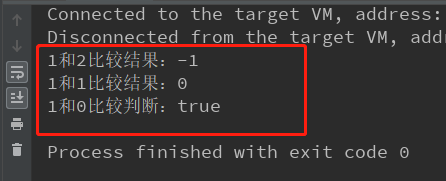5、BigDecimal保留两位小数及舍入模式
public BigDecimal setScale(int newScale, int roundingMode)

用于格式化小数的方法，第一个值表示保留几位小数，第二个值表示格式化的类型。 8种类型如下：
格式化类型描述ROUND_DOWN舍弃多余位数，如1.55会格式化为1.5，-1.55会格式化为-1.5ROUND_UP进位处理，如1.52会格式化为1.6，-1.52会格式化为-1.6ROUND_HALF_UP四舍五入，如果舍弃部分>= .5，则进位ROUND_HALF_DOWN五舍六入，如果舍弃部分> .5，则进位ROUND_CEILING正无穷大方向舍入模式。如果值为正数，则与ROUND_UP模式相同；如果值为负数，则与ROUND_DOWN模式相同ROUND_FLOOR负无穷大方向舍入模式。如果值为正数，则与ROUND_DOWN模式相同；如果值为负数，则与ROUND_UP模式相同ROUND_UNNECESSARY确认值的小数位数是否与传入第一个参数（保留小数的位数）相等，如果符合则返回值，如果不符抛出异常ROUND_HALF_EVEN如果舍弃部门左边的数字为奇数，则与ROUND_HALF_UP模式相同，如果为偶数则与ROUND_HALF_DOWN模式相同
代码示例：
public static void main(String[] args) {
BigDecimal a = BigDecimal.valueOf(5.445);
System.out.println("5.445舍弃多余位数：" + a.setScale(2, BigDecimal.ROUND_DOWN));
System.out.println("5.445进位处理：" + a.setScale(2, BigDecimal.ROUND_UP));
System.out.println("5.445四舍五入(舍弃部分>= .5,进位)：" + a.setScale(2, BigDecimal.ROUND_HALF_UP));
System.out.println("5.445四舍五入(舍弃部分未> .5,舍弃)：" + a.setScale(2, BigDecimal.ROUND_HALF_DOWN));
System.out.println("5.446四舍五入(舍弃部分> .5,进位)：" + BigDecimal.valueOf(5.446).setScale(2, BigDecimal.ROUND_HALF_DOWN));
}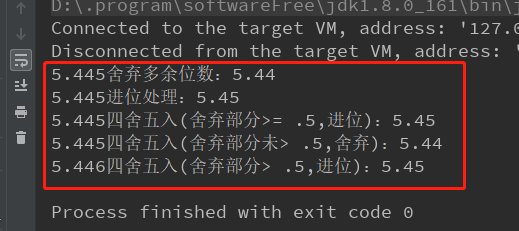6、BigDecimal其他方法及常量
代码类型描述BigDecimal.ZERO常量初始化一个为0的BigDecimal对象BigDecimal.ONE常量初始化一个为1的BigDecimal对象BigDecimal.TEN常量初始化一个为10的BigDecimal对象public BigDecimal abs()方法求绝对值，不管正数还是负数，都得到正数public BigDecimal negate()方法求相反数，正变负，负变正public BigDecimal pow(int n)方法求乘方，如BigDecimal.valueOf(2).pow(3)的值为8public BigDecimal max(BigDecimal val)方法两值比较，返回最大值public BigDecimal min(BigDecimal val)方法两值比较，返回最小值
展开全文java 后端
• BigDecimal decimal = new BigDecimal("1.12345"); System.out.println(decimal); BigDecimal setScale = decimal.setScale(4,BigDecimal.ROUND_HALF_DOWN); System.out.println(setScale);
• 作者：RaphetS第一种方法使用DecimalFormat类举个例子，假如我们需要保留两位小数，我们可以这样写DecimalFormat df = new DecimalFormat("0.00");测试如下：double d = 0.200;DecimalFormat df = new DecimalFormat...
• bigdecimal保留两位小数 public class test1_format { public static void main(String[] args) { BigDecimal decimal = new BigDecimal(“1.12345”); System.out.println(decimal); BigDecimal setScale = decimal...java 人工智能 大数据 聚类
• 0.00 或者#.00 格式：小数点后两位，不足用0补足。 DecimalFormat df1 = new DecimalFormat("#.00"); System.out.println(df1.format(2.2));// 2.20 System.out.println(df1.format(2.246));// 2.25 //2...
• Kafka 的消费者如何消费数据 消费者负载均衡策略 数据有序 kafaka 生产数据时数据的分组策略 复习学习必备44个Kafka知识（基础+进阶+高级） Kafka基础篇知识（17） Kafka进阶篇知识（15） Kafka高级篇知识...java 经验分享
• java代码-BigDecimal 四舍五入保留2位小数. 金额从单位分转成单位元代码
• 问题：数据库中的数据为1.23456---------对应的----------java属性为Bigdecimal类型，在页面中显示时只保留小数点后两位，应该怎么做呢。 答案1：一部人因为经常用Bigdecimal用+-*/的精准运算，就会用如以下的工具...
• return ((BigDecimal) value).setScale( 2 ).toString(); } return value; } } JSON转换 JSONObject.toJSONString(object, bigDecimalValueFilter,SerializerFeature.WriteNullNumberAsZero); ...
• BigDecimal保留两位小数及格式化成百分比在项目中经常会用到小数的一些计算，而float和double类型的主要设计目标是为了科学计算和工程计算。他们执行二进制浮点运算，这是为了在广域数值范围上提供较为精确的快速...
• 使用java.math.BigDecimal工具类实现 java保留两位小数问题： 方式一(四舍五入形式保留两位小数，注意模式ROUND_HALF_UP)： 四舍五入 double f = 111231.5585; BigDecimal...
• 原创： 蜀中亮子 玄说前端 小数运算的问题在 js 中的小数运算中，一直存在着一个问题，比如：0.1+0.2=0.30000000000000004 、0.4-0.3=0.10000000000000003。那么为什么会出现这种情况呢？这种情况又如何解决呢？为...
• 在Jackson依赖中下使用 导入依赖 ... } } } } 保留位小数删掉一个0即可 三位小数同上java
• **问题：**将两个long类型的数相除后转换为BigDecimal类型并保留两位小数。 思路： 1.先将long转换为double类型再相除 2.相除之后再转换为BigDecimal类型 3.最后是设置小数位数，并设置两位小数后面的数的处置方式。...java
• public static void main(String[] args) { BigDecimal t1 = new BigDecimal("3.15289"); BigDecimal t2 = new BigDecimal("-3.11289"); // 新的t1值，并初始化 BigDecimal newt...
• double类型转bigdecimal保留两位小数，小数部分不足时补0 public static void main(String[] args){ //保留4位小数，#.0000或0.0000都可以，#.####或0.####是入参必须要有四位小数才可以使用。 DecimalFormat ...java
• BigDecimal a = new BigDecimal(500.22); //保留两位小数 BigDecimal b = a .setScale(2, RoundingMode.HALF_UP);java
• ## BigDecimal保留小数位

千次阅读 2017-10-25 20:45:56
package com.algorithms;import java.math.BigDecimal; import java.util.Scanner;/** * 调和级数求和 * @author LYY * */ public class TiaoHeJiShuTest { public static void main(String[] args) { Systemjava
• 撰文：王国义审校：叔宇1、意义小数的除法是已知个因数的积与其中一个因数，求另一个因数的运算，这和整数的除法一致。2、计算方法 ①除数是整数的除法：按整数除法的计算方法，商的小数点要和被除数的小数点对齐...
• 测试代码如下： double dayDiff2 = 5.06135778; BigDecimal usedM = new BigDecimal(dayDiff2);... //保留两位小数且四舍五入 usedM = usedM.setScale(2, BigDecimal.ROUND_HALF_UP); 测试结果如图： ...
• //保留两位小数并展示千分位符 DecimalFormat df1 = new DecimalFormat("##,##0.00"); System.out.println(df1.format(0.2));// 0.20 System.out.println(df1.format(0.235));// 0.24 System.out.println(df1....java...